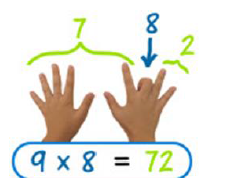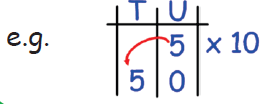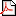Top Tips to Learn Times Table Easily From 1 to 15 Times TableWhy are times tables important?

We would be amazed at how much of our maths at school and in real life is based on TABLES.It is important that your child knows all of their times tables (as much possible).How to learn 2 Times Table2X is just doubling the number. The same as adding the number to itself.

 1 x 2 = 2 5 x 2 = 10 9 x 2 = 18 2 x 2 = 4 6 x 2 = 12 10 x 2 = 20 3 x 2 = 6 7 x 2 = 14 11 x 2 = 22 4 x 2 = 8 8 x 2 = 16 12 x 2 = 24How to learn 3 Times TableThere is a clever trick to find out if a number is in the 3x tables. If the digits in the number add up to either 3, 6 or 9, then that number is in the 3x tables.

e.g. 27 2 + 7 = 9

 1 x 3 = 3 5 x 3 = 15 9 x 3 = 27 2 x 3 = 6 6 x 3 = 18 10 x 3 = 30 3 x 3 = 9 7 x 3 = 21 11 x 3 = 33 4 x 3 = 12 8 x 3 = 24 12 x 3 = 36How to learn 4 Times Table4x simply double the number and double it again. Notice how, in the 4x tables, all of the units are even digits.

 1 x 4 = 4 5 x 4 = 20 9 x 4 = 36 2 x 4 = 8 6 x 4 = 24 10 x 4 = 40 3 x 4 = 12 7 x 4 = 28 11 x 4 = 44 4 x 4 = 16 8 x 4 = 32 12 x 4 = 48How to learn 5 Times Table5x has a pattern: 5, 10, 15, 20, etc. So, numbers in the 5 x tables
always end in either 0 or 5
Or, you could x10 and half

 1 x 5 = 5 5 x 5 = 10 9 x 5 = 45 2 x 5 = 10 6 x 5 = 30 10 x 5 = 50 3 x 5 = 15 7 x 5 = 35 11 x 5 = 55 4 x 5 = 20 8 x 5 = 40 12 x 5 = 60How to learn 6 Times Table6x remember to use the facts that you have already learned. 6 x 4 = 24 so 4 x 6 = 24.
6 x 6 = 36. Notice how that rhymes!
6 x 8 = 48. That rhymes too!

 1 x 6 = 6 5 x 6 = 30 9 x 6 = 54 2 x 6 = 12 6 x 6 = 36 10 x 6 = 60 3 x 6 = 18 7 x 6 = 42 11 x 6 = 66 4 x 6 = 24 8 x 6 = 48 12 x 6 = 72How to learn 7 Times Table7x remember to use the facts that you have already learnt.
7 x 5 = 35 so 5 x 7 =35

 1 x 7 = 7 5 x 7 = 35 9 x 7 = 63 2 x 7 = 14 6 x 7 = 42 10 x 7 = 70 3 x 7 = 21 7 x 7 = 49 11 x 7 = 77 4 x 7 = 28 8 x 7 = 56 12 x 7 = 84How to learn 8 Times Table8x all of the numbers in the 8 times tables are even.
Can you spot the pattern? The ones digits go down in 2s (8, 6, 4, 2, 0)

 1 x 8 = 8 5 x 8 = 40 9 x 8 = 72 2 x 8 = 16 6 x 8 = 48 10 x 8 = 80 3 x 8 = 24 7 x 8 = 56 11 x 8 = 88 4 x 8 = 32 8 x 8 = 64 12 x 8 = 96How to learn 9 Times Table9x has a pattern: 9, 18, 27, 36, 45, 54, 63, 72, 81, 90
Notice how the ones go down: 9,8,7,6, ...? And the tens go up: 1,2,3,...? Your hands can help! Example: to multiply 9 by 8: hold your 8th finger down, and you can count "7" and "2" ... the answer is 72.1 x 9 = 9 5 x 9 = 45 9 x 9 = 81 2 x 9 = 18 6 x 9 = 54 10 x 9 = 90 3 x 9 = 27 7 x 9 = 63 11 x 9 = 99 4 x 9 = 36 8 x 9 = 72 12 x 9 = 108How to learn 10 Times Table10x is maybe the easiest of them all ... just move your digit one space to the left and add a zero as a place holder.1 x 10 = 10 5 x 10 = 50 9 x 10 = 90 2 x 10 = 20 6 x 10 = 60 10 x 10 = 100 3 x 10 = 30 7 x 10 = 70 11 x 10 = 110 4 x 10 = 40 8 x 10 = 80 12 x 10 = 120How to learn 11 Times Table11x is easy-peasy up to 9 x 11: just write the number that you are multiplying twice. E.g. 3 x 11 = 33

 1 x 11 = 11 5 x 11 = 55 9 x 11 = 99 2 x 11 = 22 6 x 11 = 66 10 x 11 = 110 3 x 11 = 33 7 x 11 = 77 11 x 11 = 121 4 x 11 = 44 8 x 11 = 88 12 x 11 = 132How to learn 12 Times Table12x Note that 12 is 10 + 2, so 12 x something is 10 x something + 2 x something.
Example: 4 x 12 =
10 x 4 = 40 + 2 x 4 = 8
40 + 8 = 48

 1 x 12 = 12 5 x 12 = 60 9 x 12 = 108 2 x 12 = 24 6 x 12 = 72 10 x 12 = 120 3 x 12 = 36 7 x 12 = 84 11 x 12 = 132 4 x 12 = 48 8 x 12 = 96 12 x 12 = 144How to learn 15 Times Table15x Numbers in the 15 times tables will always end in a 5 or a 0.
Note that 15 is 10 + 5, so 15 x something is 10 x something + 5 x something.
Example: 6 x 15 =
10 x 6 = 60 + 5 x 6 = 30
60 + 30 = 90

 1 x 15 = 15 5 x 15 = 75 9 x 15 = 135 2 x 15 = 30 6 x 15 = 90 10 x 15 = 150 3 x 15 = 45 7 x 15 = 105 11 x 15 = 165 4 x 15 = 60 8 x 15 = 120 12 x 15 = 1802-times-table-1-to-60.pdf 45 Kb 27/02/173-times-table-1-to-60.pdf 45 Kb 27/02/174-times-table-1-to-60.pdf 45 Kb 27/02/175-times-table-1-to-60.pdf 45 Kb 27/02/176-times-table-1-to-60.pdf 45 Kb 27/02/177-times-table-1-to-60.pdf 45 Kb 27/02/178-times-table-1-to-60.pdf 45 Kb 27/02/179-times-table-1-to-60.pdf 45 Kb 27/02/1710-times-table-1-to-60.pdf 45 Kb 27/02/17

Times Table Challenge:bronze-times-table-challenge.pdf 29 Kb 27/02/17silver-times-table-challenge.pdf 29 Kb 27/02/17gold-times-table-challenge.pdf 29 Kb 27/02/17platinum-times-table-challenge.pdf 33s Kb 27/02/17

What math times time do you want to generate ?• When your child has begun to learn a table, practise the table for 5 minutes each day with them.
• Ask your child to chant each times table out loud: Example: "two" times "two" is "four".
• Make a Rhyme
• It is very important to say the whole table, not just the answers, again and again!
• Break down each table into manageable chunks. For example, ask them 1 x 3, 2 x 3 and 5 x 3 until they know the answers. Then add the next one
• Work on pairs of tables, Example if your kid is learning the 2 times table they can use their doubling facts to calculate the 4 times tables.
• Test your child by asking questions at them, out of order reminding them that they can use facts that they are confident with to work out trickier ones.Example if they know 2 x 6=12 just double to find 4x6.
• Keep checking that they still know the facts they have learnt and revisit previously learnt facts
• Encourage your child to write out the table they are learning again and again.Times Table Tricks 2 to 11►

2 Times Table Trick

4 Times Table Trick

5 Times Table Trick

6 & 7 Times Table Trick

8 Times Table TrickTop Calculators ►

Tax Calculator, Calories Burned Calculator, PERT, SD Calculator, Dog Age Calculator, Children Equal Playing Time Calculator